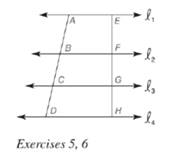Chapter 5.6, Problem 6EElementary Geometry For College St...

7th Edition
Alexander + 2 others
ISBN: 9781337614085

Solutions

Chapter
SectionElementary Geometry For College St...

7th Edition
Alexander + 2 others
ISBN: 9781337614085
Textbook Problem

Given: l 1 ∥ l 2 ∥ l 3 ∥ l 4 , A B = 7 , B C = 5 , C D = 4 , E F = 6 Find: F G , G H , E HTo determine

To find:

FG,GH,EH.

Explanation

Given:

l1l2l3l4 and AB=7,BC=5,CD=4,EF=6.

Theorem used:

When three (or more) parallel lines are cut by a pair of transversals, the transversals are divided proportionally by the parallel lines.

Calculation:

Given: l1l2l3l4

Since when three (or more) parallel lines are cut by a pair of transversals, the transversals are divided proportionally by the parallel lines.

ABEF=BCFG=CDGH

Also given that AB=7,BC=5,CD=4,EF=6.

Thus 76=5FG=4GH.

Consider 76=5FG

FG=567=307=427

Now consider 76=4GH

Still sussing out bartleby?

Check out a sample textbook solution.

See a sample solution

The Solution to Your Study Problems

Bartleby provides explanations to thousands of textbook problems written by our experts, many with advanced degrees!

Get Started

In Exercises 4756, solve the given equation for the indicated variable. 27=32x1

Finite Mathematics and Applied Calculus (MindTap Course List)

Evaluate the limit, if it exists. limh0(3+h)131h

Single Variable Calculus: Early Transcendentals

For y = x3 ln x, y = a) x2(1 + 3 ln x) b) x3 + 3x2 (ln x) c) 3x d) 4x2(ln x)

Study Guide for Stewart's Single Variable Calculus: Early Transcendentals, 8th